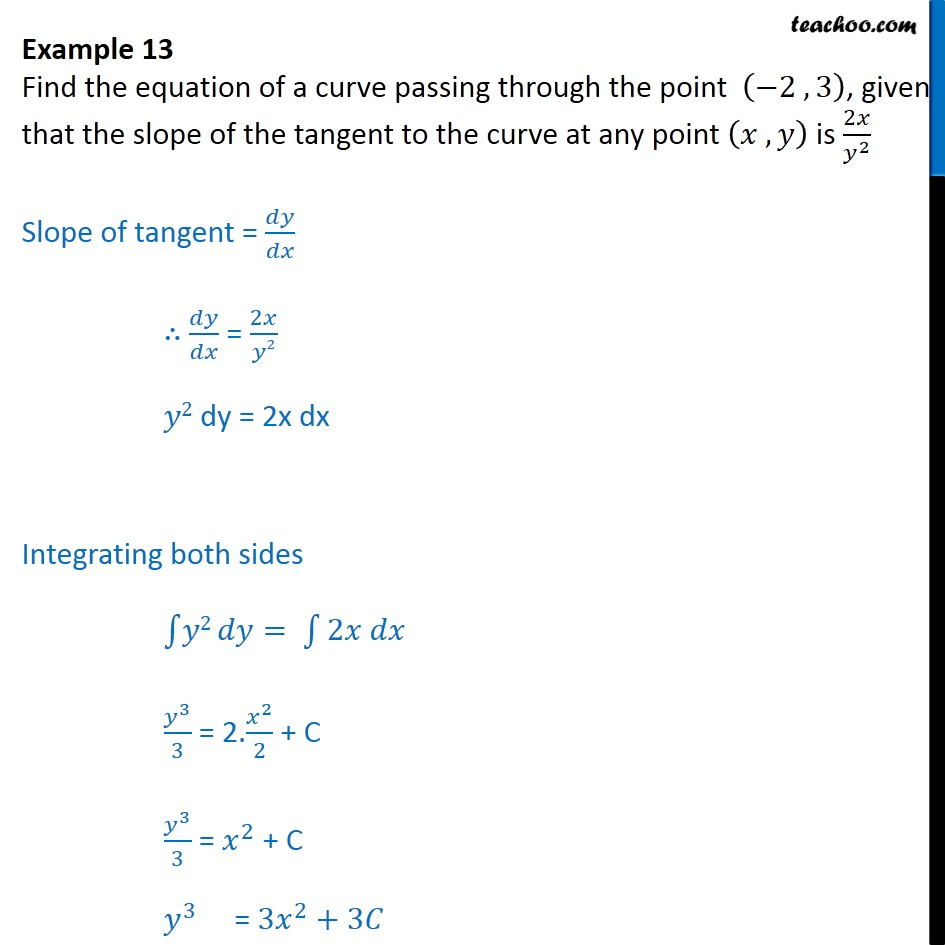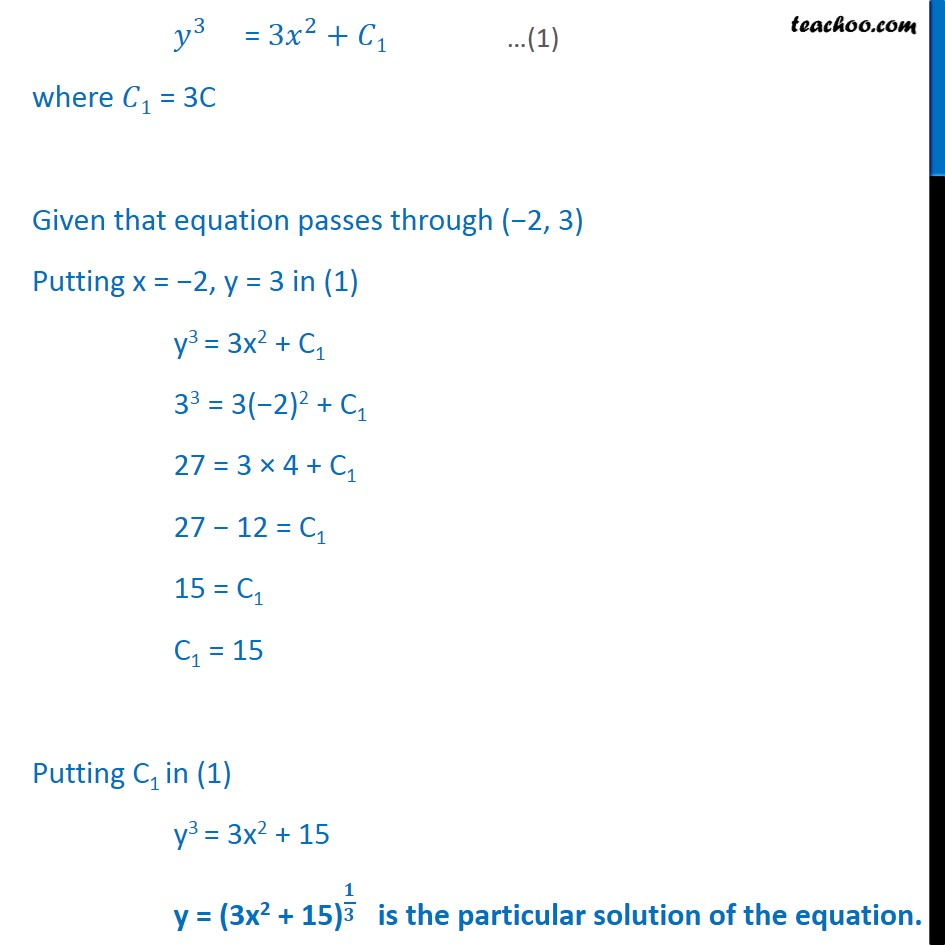Variable separation - Statement given

Chapter 9 Class 12 Differential Equations
Concept wiseIntroducing your new favourite teacher - Teachoo Black, at only ₹83 per month

### Transcript

Example 13 Find the equation of a curve passing through the point (−2 ,3), given that the slope of the tangent to the curve at any point (𝑥 , 𝑦) is 2𝑥/𝑦^2 Slope of tangent = 𝑑𝑦/𝑑𝑥 ∴ 𝑑𝑦/𝑑𝑥 = 2𝑥/𝑦2 𝑦2 dy = 2x dx Integrating both sides ∫1▒𝑦2 𝑑𝑦= ∫1▒〖2𝑥 𝑑𝑥〗 𝑦^3/3 = 2.𝑥^2/2 + C 𝑦^3/3 = 𝑥^2 + C 𝑦^3 = 〖3𝑥〗^2+3𝐶 𝑦^3 = 〖3𝑥〗^2+𝐶1 where 𝐶1 = 3C Given that equation passes through (−2, 3) Putting x = −2, y = 3 in (1) y3 = 3x2 + C1 33 = 3(−2)2 + C1 27 = 3 × 4 + C1 27 − 12 = C1 15 = C1 C1 = 15 Putting C1 in (1) y3 = 3x2 + 15 y = "(3x2 + " 〖"15)" 〗^(𝟏/𝟑) " "is the particular solution of the equation.